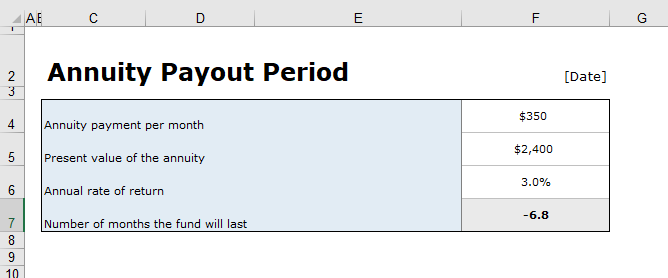# Annuity Payout Calculator

After choosing the option of purchasing the annuity for securing the future, every person takes interest in the amount of payout he will receive each month or year. When you pay a lump sum, you get the payment each month in series. This type of investment is known as an annuity.

## What is the annuity payout calculator?

An annuity payout calculator is a tool that is used for estimating the amount of annuity payout. The length of the annuity payout can be fixed or variable. Whether the payout is fixed or changes with time, the length of payout can be calculated using the calculator.

### Why is the annuity payout calculator used?

There are lots of concerns about a person when it comes to buying the annuity. People may want to use the annuity payout calculator because of the following reasons:

1. How much there will be a reduction in the amount that will be received each month
2. How much amount is required to be invested in order to receive the desired amount of payment?
3. The number of years you will have to make the investment.

### Annuity payout calculator template:

This calculator is preferable to be used in order to calculate the payments from the monthly expenses or the value of ROI. All you need to do is input the details in the calculator. It will calculate the result automatically. The annuity payout calculator template is a useful tool since it enables the user to save his time and energy on the tedious task of calculating the annuity payout of any length. This template enables the user to select the best action to take or the best process to go through in order to receive the annuity payout.

A lot of websites provide a variety of tools including the annuity payout calculator for an accurate and error-free calculation of the annuity payout. Some of the calculators are very simple that ask the user to provide some very basic details for the calculation of payout. Although there are also some very complex calculators available, you can opt for a simple calculator if you don’t have numerous variables to consider for calculation.

#### How to use the annuity payout calculator?

Using the annuity payout calculator is a simple task. Here are a few steps that can be followed in order to make the effective use of this calculator.

1. Provide the amount to a calculator that you want to withdraw
2. Mention the intervals after which you will want to withdraw the money
3. Mention the amount of principal at the start of the withdrawal
4. Mention the annual growth rate in the calculator
5. Length of annuity should also be mentioned since the result obtained depends on it

It is important to fill in all the fields of the calculator that it requires to be filled. The calculator uses a formula for the calculation of annuity payout. If you don’t mention any of the important details that your calculator asks you to provide, you will not be able to get the right calculations.

PreviewFormat: MS Excel [.xls & .xlsx]